## Triangle Inscribing in a CircleSelect three points at random on a unit Circle. Find the distribution of possible areas. The first point can be assigned coordinates (1, 0) without loss of generality. Call the central angles from the first point to the second and thirdand. The range ofcan be restricted to [0,] because of symmetry, butcan range from. Then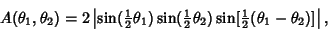(1)

so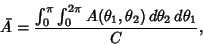(2)

where(3)

Therefore,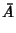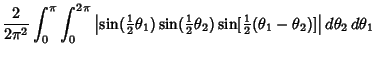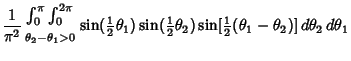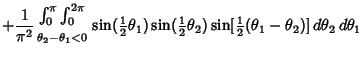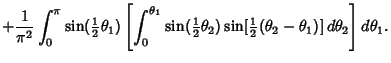(4)

But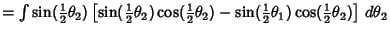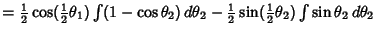(5)

Write (4) as(6)

then(7)

and(8)

From (6),(9)

so(10)

Also,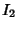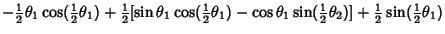(11)

so(12)

Combining (10) and (12) gives(13)

The Variance is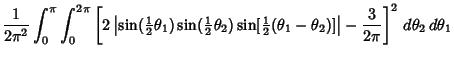(14)